# SSC CGL 2018 Practice Test Papers | Reasoning Ability (Day-42)

Dear Aspirants, Here we have given the Important SSC CGL Exam 2018 Practice Test Papers. Candidates those who are preparing for SSC CGL 2018 can practice these questions to get more confidence to Crack SSC CGL 2018 Examination.

[WpProQuiz 3309]

Click “Start Quiz” to attend these Questions and view Explanation

1) If ‘L’ means ‘×’, ‘m’ means ‘÷’, ‘P’ means ‘+’, and ‘Q’ means ‘–’. Then find out the value of the equation ?

24 M 8 P 6 Q 1 6 L 3 L 3 = ?

a) 135

b) 145

c) 155

d) –135

2) A walks 10 km towards north. From there he turned 180o clockwise and walks for 6 km. Then he takes left turn and walks 3 km. How far and in which direction is he from his starting point?

a) 5 km, North-East

b) 7 km, East

c) 5 km, East

d) 7 km, West

3) In a row A is 7th from the left and B is 9thfrom the right. If both interchange their places, then A becomes 11th from the left. The number of persons in the row is :

a) 18

b) 20

c) 21

d) 19

Direction (4-6):
The statement below is followed by two conclusions labelled I and II. Assuming that the

Information in the statement is true, which conclusion(s) logically and definitely follow(s) from the information given in the statement.

4) Statement:

Some Glasses are Mirror

Some mirror is not Plate

All plate is vessel

Conclusion :

1. Some vessel is Glass in a possibility
2. Some Plates are never Glasses

a) Only I follow

b) Only II follow

c) Both follow

d) None follow

5) Statement:

Some Glasses are Mirror

Some mirror is not Plate

All plate is vessel

Conclusion:

1. Some vessel is not Mirror
2. Some Plates are Glass

a) Only I follow

b) Only II follow

c) Both follow

d) None follow

6) Statement

Some Glasses are Mirror

Some mirror is not Plate

All plate is vessel

Conclusion:

1. All mirrors are Plates
2. Some mirrors are not plates

a) Only I follow

b) Only II follow

c) Either I or II follow

d) Neither I nor II follow

7) Choose the correct alternative from the given ones that will complete the series.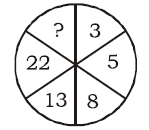a) 29

b) 39

c) 38

d) 45

8) From the given alternative words, select the word which cannot be formed using the letters of the given word:

MEMORANDUM

a) RAM

b) ROUND

c) MARE

d) ORANGE

9) Which one set of letters when sequentially placed at the gaps in the given letter series shall complied it ?

ab _ ctmoh _ bbct _ ohabb _

a) b a m h

b) a b m h

c) h c a b

d) c a h b

10) Select the related word/letters/number from the given alternatives

12:30::40:?

a) 90

b) 100

c) 120

d) 150

After changing the sign

24 ÷ 8 + 6 – 16 × 3 × 3

= 3 + 6 – 144

= 9 – 144 = – 135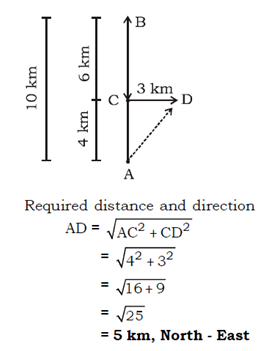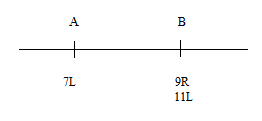Number of persons in the row=11+9 – 1 =19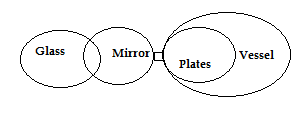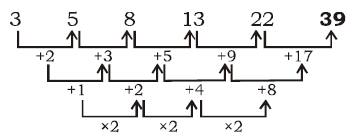Word ORANGE cannot be formed since G is not there in the word.

a b b/ c t m o h / a b b / c t mo h / a b b h